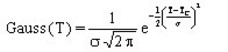# Volumetric heat capacity, constant + Gaussian of T

## Presentation

This model defines a volumetric heat capacity ρCp as a constant quantity, taking the energy of phase transition (Curie point, ...) into consideration.

## Mathematical model

The volumetric heat capacity is the combination of a constant quantity and a Gaussian function of the temperature.

The corresponding mathematical formula is written:

ρCp = ρCpa +E.Gauss(T) withwhere:

• ρCpa is a constant quantitythe volumetric heat capacity of a material at T = 0 °C (J/m3/°C)
• E is the energy of the phase transition (J/m3)
• σ is the typical Gaussian standard deviation (°C)
• TC is transition temperature (Curie temperature, …) (°C)

The shape of ρCP(T) curve is presented in the figure below.